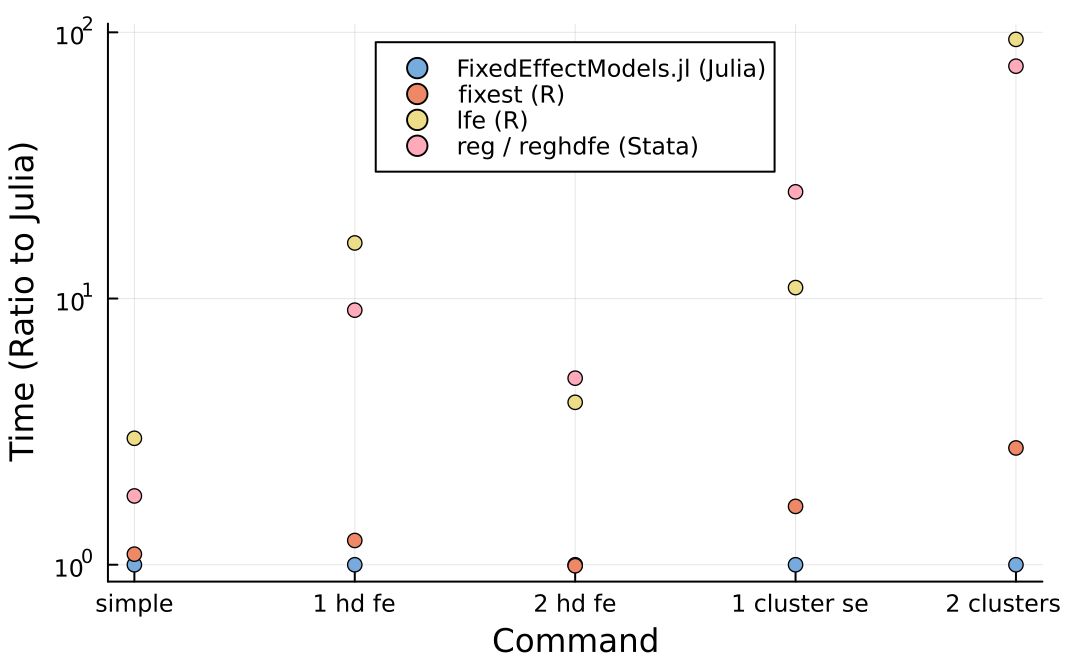# FixedEffectModels

0

0

0

17

### contributorsThis package estimates linear models with high dimensional categorical variables and/or instrumental variables.

## Installation

The package is registered in the `General` registry and so can be installed at the REPL with `] add FixedEffectModels`.

## Benchmarks

The objective of the package is similar to the Stata command `reghdfe` and the R function `felm`. The package tends to be much faster than these two options.Performances are roughly similar to the newer R function `feols` (note: use `tol = 1e-6, drop_singletons = false` to match the default options of `feols`). The main difference is that `FixedEffectModels` can also run the demeaning operation on a GPU (with `method = :gpu`).

## Syntax

``````using DataFrames, RDatasets, FixedEffectModels
df = dataset("plm", "Cigar")
reg(df, @formula(Sales ~ NDI + fe(State) + fe(Year)), Vcov.cluster(:State), weights = :Pop)
# =====================================================================
# Number of obs:               1380   Degrees of freedom:            32
# R2:                         0.803   R2 Adjusted:                0.798
# F Statistic:              13.3382   p-value:                    0.001
# R2 within:                  0.139   Iterations:                     6
# Converged:                   true
# =====================================================================
#         Estimate  Std.Error  t value Pr(>|t|)   Lower 95%   Upper 95%
# ---------------------------------------------------------------------
# NDI  -0.00526264 0.00144097 -3.65216    0.000 -0.00808942 -0.00243586
# =====================================================================
``````
• A typical formula is composed of one dependent variable, exogeneous variables, endogeneous variables, instrumental variables, and a set of high-dimensional fixed effects.

``````dependent variable ~ exogenous variables + (endogenous variables ~ instrumental variables) + fe(fixedeffect variable)
``````

High-dimensional fixed effect variables are indicated with the function `fe`. You can add an arbitrary number of high dimensional fixed effects, separated with `+`. You can also interact fixed effects using `&` or `*`.

For instance, to add state fixed effects use `fe(State)`. To add both state and year fixed effects, use `fe(State) + fe(Year)`. To add state-year fixed effects, use `fe(State)&fe(Year)`. To add state specific slopes for year, use `fe(State)&Year`. To add both state fixed-effects and state specific slopes for year use `fe(State)*Year`.

``````reg(df, @formula(Sales ~ Price + fe(State) + fe(Year)))
reg(df, @formula(Sales ~ NDI + fe(State) + fe(State)&Year))
reg(df, @formula(Sales ~ NDI + fe(State)&fe(Year)))              # for illustration only (this will not run here)
reg(df, @formula(Sales ~ (Price ~ Pimin)))
``````

To construct formula programatically, use

``````reg(df, term(:Sales) ~ term(:NDI) + fe(:State) + fe(:Year))
``````
• Standard errors are indicated with the prefix `Vcov` (with the package Vcov)

``````Vcov.robust()
Vcov.cluster(:State)
Vcov.cluster(:State, :Year)
``````
• The option `weights` specifies a variable for weights

``````weights = :Pop
``````
• The option `contrasts` specifies particular contrasts for a dummy variable in the formula, e.g.

``````reg(df, @formula(Sales ~ Year); contrasts = Dict(:Year => DummyCoding(base = 80)))
``````
• The option `save` can be set to one of the following: `none` (default) to save nothing `:residuals` to save residuals, `:fe` to save fixed effects. You can access the result with `residuals()` and `fe()`

-

• The option `method` can be set to one of the following: `:cpu`, `:gpu` (see Performances below).

## Output

`reg` returns a light object. It is composed of

• the vector of coefficients & the covariance matrix (use `coef`, `coefnames`, `vcov` on the output of `reg`)
• a boolean vector reporting rows used in the estimation
• a set of scalars (number of observations, the degree of freedoms, r2, etc)
• with the option `save = true`, a dataframe aligned with the initial dataframe with residuals and, if the model contains high dimensional fixed effects, fixed effects estimates (use `residuals` or `fe` on the output of `reg`)

Methods such as `predict`, `residuals` are still defined but require to specify a dataframe as a second argument. The problematic size of `lm` and `glm` models in R or Julia is discussed here, here, here here (and for absurd consequences, here and there).

You may use RegressionTables.jl to get publication-quality regression tables.

## Performances

By default, `FixedEffectModels` uses as many threads as `Threads.nthreads()`. Use the option `nthreads` to select the number of threads to use in the estimation. Default to `Threads.nthreads()`.

The package has support for GPUs (Nvidia) (thanks to Paul Schrimpf). This can make the package an order of magnitude faster for complicated problems.

To use GPU, run `using CUDA` before `using FixedEffectModels`. Then, estimate a model with `method = :gpu`. For maximum speed, set the floating point precision to `Float32` with `double_precision = false`.

``````using CUDA, FixedEffectModels
df = dataset("plm", "Cigar")
reg(df, @formula(Sales ~ NDI + fe(State) + fe(Year)), method = :gpu, double_precision = false)
``````

## Solution Method

Denote the model `y = X β + D θ + e` where X is a matrix with few columns and D is the design matrix from categorical variables. Estimates for `β`, along with their standard errors, are obtained in two steps:

1. `y, X` are regressed on `D` using the package FixedEffects.jl
2. Estimates for `β`, along with their standard errors, are obtained by regressing the projected `y` on the projected `X` (an application of the Frisch Waugh-Lovell Theorem)
3. With the option `save = true`, estimates for the high dimensional fixed effects are obtained after regressing the residuals of the full model minus the residuals of the partialed out models on `D` using the package FixedEffects.jl

# References

Baum, C. and Schaffer, M. (2013) AVAR: Stata module to perform asymptotic covariance estimation for iid and non-iid data robust to heteroskedasticity, autocorrelation, 1- and 2-way clustering, and common cross-panel autocorrelated disturbances. Statistical Software Components, Boston College Department of Economics.

Correia, S. (2014) REGHDFE: Stata module to perform linear or instrumental-variable regression absorbing any number of high-dimensional fixed effects. Statistical Software Components, Boston College Department of Economics.

Fong, DC. and Saunders, M. (2011) LSMR: An Iterative Algorithm for Sparse Least-Squares Problems. SIAM Journal on Scientific Computing

Gaure, S. (2013) OLS with Multiple High Dimensional Category Variables. Computational Statistics and Data Analysis

04/12/2021

29 days ago

448 commits